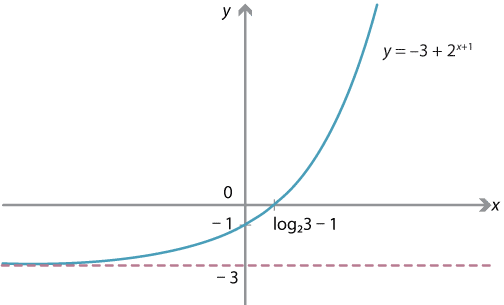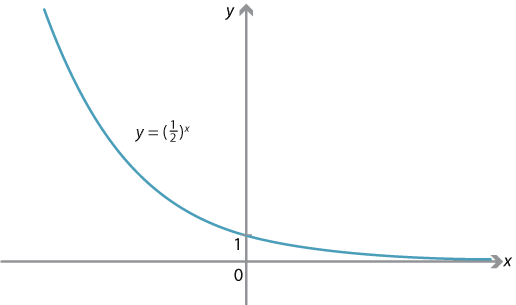## Content

### Graphing exponential functions

Having seen the general shape of the graphs $$y = a^x$$, we can graph related functions, including those obtained by transformations such as dilations, reflections or translations.

Let us first revise these transformations; for more details, we refer to the module Functions II.

• A dilation in the $$x$$-direction from the $$y$$-axis with factor $$k$$ maps $(x,y) \mapsto (kx,y).$ The plane is stretched out horizontally from the $$y$$-axis by a factor of $$k$$.
• A dilation in the $$y$$-direction from the $$x$$-axis with factor $$k$$ maps $(x,y) \mapsto (x,ky).$ The plane is stretched vertically from the $$x$$-axis by a factor of $$k$$.
• The reflection in the $$y$$-axis maps $(x,y) \mapsto (-x,y).$ The plane is reflected horizontally in the vertical axis: everything in the plane on one side of the $$y$$-axis goes to its mirror image on the other side of the $$y$$-axis.
• The reflection in the $$x$$-axis maps $(x,y) \mapsto (x,-y).$ The plane is reflected vertically in the horizontal axis.
• A translation in the $$x$$-direction by $$a$$ units maps $(x,y) \mapsto (x+a, y).$ If $$a>0$$, the translation is to the right, and if $$a<0$$, the translation is to the left.
• A translation in the $$y$$-direction by $$a$$ units maps $(x,y) \mapsto (x, y+a).$ If $$a>0$$, the translation is upwards, and if $$a<0$$, the translation is downwards.

Note that, as the $$x$$-axis is an asymptote for the graph $$y=a^x$$, any graph obtained by dilating, reflecting or translating such a graph will also have an asymptote.

Note also that, for any $$a>0$$ with $$a \neq 1$$, the function $$y=a^x$$ has no critical points: the derivative $$\dfrac{dy}{dx} = \log_e a \cdot a^x$$ is never 0. A graph obtained from $$y=a^x$$ by dilations, reflections in the axes and translations also has no critical points.

#### Example

Sketch the graph of $$y = 2^{x+1}-3$$.

#### Solution

This equation can be written as $$y=-3 + g(x+1)$$ where $$g(x) = 2^x$$. Hence the graph is obtained from $$y=2^x$$ by successively performing the following transformations:

• translation by 1 in the negative $$x$$-direction, which gives the graph of $$y=2^{x+1}$$
• translation by 3 in the negative $$y$$-direction, which gives the graph of $$y=2^{x+1}-3$$.

These transformations shift the asymptote to $$y=-3$$.

Substituting $$x=0$$ gives a $$y$$-intercept of $$-3+2= -1$$. Substituting $$y=0$$ gives $$2^{x+1} = 3$$, so that $$x = \log_2 3 - 1$$.

This is enough information to sketch the graph.Detailed description

Note that, using the change of base rule, we can alternatively write the $$x$$-intercept of the graph as

$\log_2 3 - 1 = \dfrac{\log_e 3}{\log_e 2} - 1.$

Exercise 14

Sketch the graph of $$y= 5 - 4 \cdot 3^{2x+1}$$.

When $$0 < a < 1$$, the graph $$y=f(x)$$ of the function $$f(x)=a^x$$ has a similar shape as for the case $$a>1$$, but now $$f(x) \to 0$$ as $$x \to \infty$$ and $$f(x) \to \infty$$ as $$x \to -\infty$$.Graph of $$f(x) = \bigl( \dfrac{1}{2} \bigr)^x$$.
Detailed description

Exercise 15

1. Explain why the graph of $$y = \bigl( \dfrac{1}{2} \bigr)^x$$ is obtained from the graph of $$y=2^x$$ by reflecting in the $$y$$-axis.
2. Hence sketch the graph of $$y = 3 - \bigl( \dfrac{1}{2} \bigr)^{x+1}$$.

Exercise 16

Consider the graph $$y=3^x$$. Explain why the following two transformations on the graph have the same effect:

• dilation in the $$y$$-direction from the $$x$$-axis with factor 9
• translation by 2 units to the left (that is, in the negative $$x$$-direction).

Next page - Content - Graphing logarithmic functions